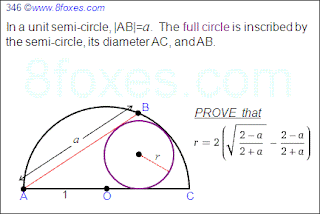## Thursday, January 12, 2012

### Fox 346

Wooow... This has been quite long time, huh? Life makes people busy (although that is not the exact excuse.)

Is everybody alive? What have been up to? Bleaug? Newzad? Polar Fox ?? ...

The following fox is a simplified version submitted by Roland Sampy. We should be able to ask a general version later. You mean next year??? Who knows hopefully earlier. Here you are...
Oh, by the way the answer was not confirmed yet. So, the claim could be wrong.A happy new year to everybody.

1.[URL=http://imageshack.us/photo/my-images/443/8foxes20121.jpg/][IMG]http://img443.imageshack.us/img443/2572/8foxes20121.jpg[/IMG][/URL]

jyu

2.http://imageshack.us/photo/my-images/443/8foxes20121.jpg/
jyu

3.2r/sqrt(4-a^2)+ar/sqrt(4-a^2) = 1+sqrt(1-2r)
(2+a)r/sqrt(4-a^2) = 1+sqrt(1-2r)
r.sqrt[(2+a)/(2-a)] = 1+sqrt(1-2r)
r.sqrt[(2+a)/(2-a)]-1 = sqrt(1-2r)
r^2.(2+a)/(2-a)-2r.sqrt[(2+a)/(2-a)]+1 = 1-2r
r(2+a)/(2-a)-2sqrt[(2+a)/(2-a)] = -2
r(2+a)/(2-a) = 2sqrt[(2+a)/(2-a)]-2
r(2+a)/(2-a) = 2[sqrt[(2+a)/(2-a)]-1]
r = 2[(2-a)/(2+a)][sqrt[(2+a)/(2-a)]-1]
r = 2[sqrt[(2-a)/(2+a)]-(2-a)/(2+a)]

jyu

4.Thank you Yu for this solution and confirming the answer. Bleaug also sent a nice one. We'll publish these later..
8foxes

5.I also got an solution, a long one and not the smartest one, but fully worked out.
See: http://home.kpn.nl/henkreuling/solutions8foxes/346_8foxes_solution.pdf

1.6.